# 共振值測量¶

## 安裝指南¶

### 接線¶

3V3 或 VCC 01 3.3v 直流（DC）電源
GND 06 地（GND）
CS（晶片選定） 24 GPIO08 (SPI0_CE0_N)
SDO 21 GPIO09 (SPI0_MISO)
SDA 19 GPIO10 (SPI0_MOSI)
SCL 23 GPIO11 (SPI0_SCLK)

An alternative to the ADXL345 is the MPU-9250 (or MPU-6050). This accelerometer has been tested to work over I2C on the RPi at 400kbaud. Recommended connection scheme for I2C:

MPU-9250 pin 樹莓派引腳 樹莓派引腳名稱
3V3 或 VCC 01 3.3v 直流（DC）電源
GND 09 地（GND）
SDA 03 GPIO02 (SDA1)
SCL 05 GPIO03 (SCL1)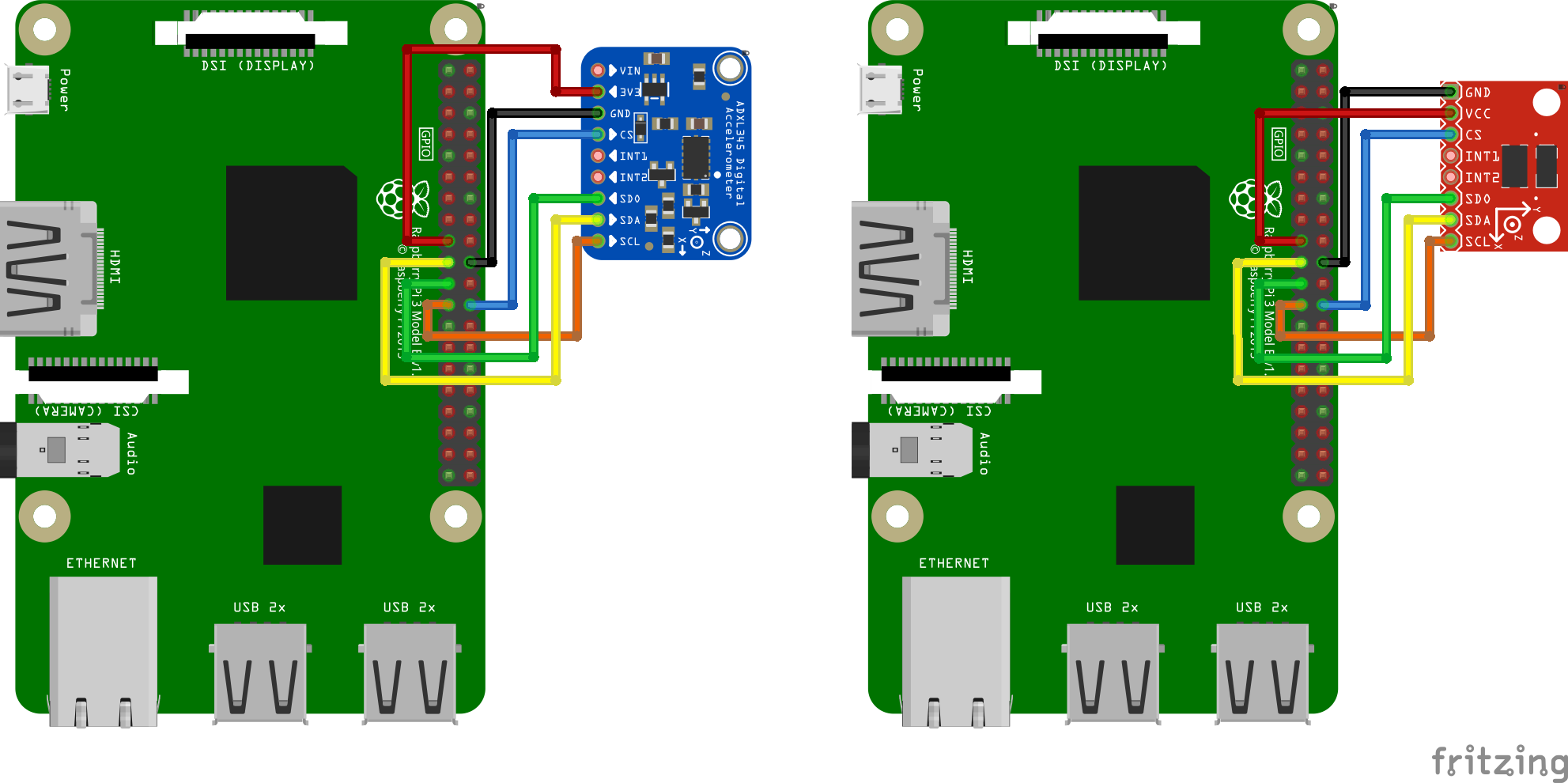### 固定加速度感測器¶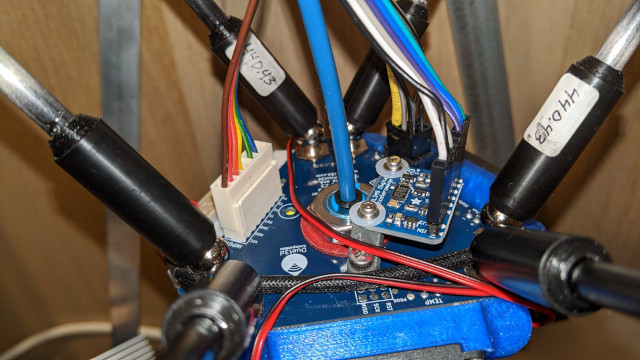### 軟體設定¶

Note that resonance measurements and shaper auto-calibration require additional software dependencies not installed by default. First, run on your Raspberry Pi the following commands:

``````sudo apt update
sudo apt install python3-numpy python3-matplotlib libatlas-base-dev
``````

Next, in order to install NumPy in the Klipper environment, run the command:

``````~/klippy-env/bin/pip install -v numpy
``````

Note that, depending on the performance of the CPU, it may take a lot of time, up to 10-20 minutes. Be patient and wait for the completion of the installation. On some occasions, if the board has too little RAM the installation may fail and you will need to enable swap.

For the ADXL345, add the following to the printer.cfg file:

``````[mcu rpi]
serial: /tmp/klipper_host_mcu

cs_pin: rpi:None

[resonance_tester]
probe_points:
100, 100, 20  # an example
``````

For the MPU-9250, make sure the Linux I2C driver is enabled and the baud rate is set to 400000 (see Enabling I2C section for more details). Then, add the following to the printer.cfg:

``````[mcu rpi]
serial: /tmp/klipper_host_mcu

[mpu9250]
i2c_mcu: rpi
i2c_bus: i2c.1

[resonance_tester]
accel_chip: mpu9250
probe_points:
100, 100, 20  # an example
``````

## 測量共振值¶

### 檢查設定¶

• 對於只有一個加速度感測器的情況，在Octoprint，輸入`ACCELEROMETER_QUERY`（遍歷已連線的加速度感測器）
• 對於「滑動床」（即有多個加速度感測器），輸入`ACCELEROMETER_QUERY CHIP=<chip>`，其中`<chip>`是設定文件中的加速度感測器命名，例如 `CHIP=bed`(參見：bed-slinger)。

``````Recv: // adxl345 values (x, y, z): 470.719200, 941.438400, 9728.196800
``````

### 測量共振值¶

``````TEST_RESONANCES AXIS=X
``````

``````[resonance_tester]
accel_per_hz: 50  # default is 75
probe_points: ...
``````

``````TEST_RESONANCES AXIS=Y
``````

``````~/klipper/scripts/calibrate_shaper.py /tmp/resonances_x_*.csv -o /tmp/shaper_calibrate_x.png
~/klipper/scripts/calibrate_shaper.py /tmp/resonances_y_*.csv -o /tmp/shaper_calibrate_y.png
``````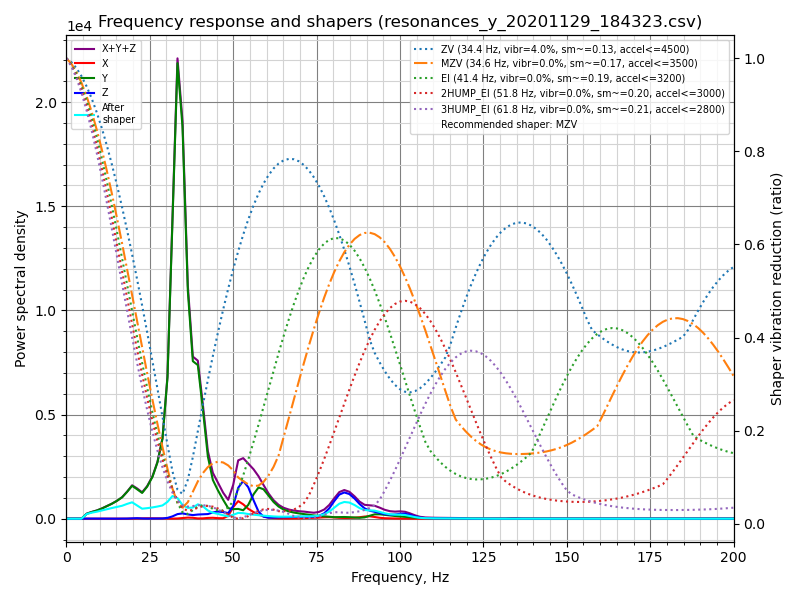``````Fitted shaper 'zv' frequency = 34.4 Hz (vibrations = 4.0%, smoothing ~= 0.132)
To avoid too much smoothing with 'zv', suggested max_accel <= 4500 mm/sec^2
Fitted shaper 'mzv' frequency = 34.6 Hz (vibrations = 0.0%, smoothing ~= 0.170)
To avoid too much smoothing with 'mzv', suggested max_accel <= 3500 mm/sec^2
Fitted shaper 'ei' frequency = 41.4 Hz (vibrations = 0.0%, smoothing ~= 0.188)
To avoid too much smoothing with 'ei', suggested max_accel <= 3200 mm/sec^2
Fitted shaper '2hump_ei' frequency = 51.8 Hz (vibrations = 0.0%, smoothing ~= 0.201)
To avoid too much smoothing with '2hump_ei', suggested max_accel <= 3000 mm/sec^2
Fitted shaper '3hump_ei' frequency = 61.8 Hz (vibrations = 0.0%, smoothing ~= 0.215)
To avoid too much smoothing with '3hump_ei', suggested max_accel <= 2800 mm/sec^2
Recommended shaper is mzv @ 34.6 Hz
``````

``````[input_shaper]
shaper_freq_x: ...
shaper_type_x: ...
shaper_freq_y: 34.6
shaper_type_y: mzv

[printer]
max_accel: 3000  # should not exceed the estimated max_accel for X and Y axes
``````

### Bed-slinger打印機¶

``````[adxl345 hotend]
# Assuming `hotend` chip is connected to an RPi
cs_pin: rpi:None

# Assuming `bed` chip is connected to a printer MCU board
cs_pin: ...  # Printer board SPI chip select (CS) pin

[resonance_tester]
# Assuming the typical setup of the bed slinger printer
probe_points: ...
``````

### 最大平滑度¶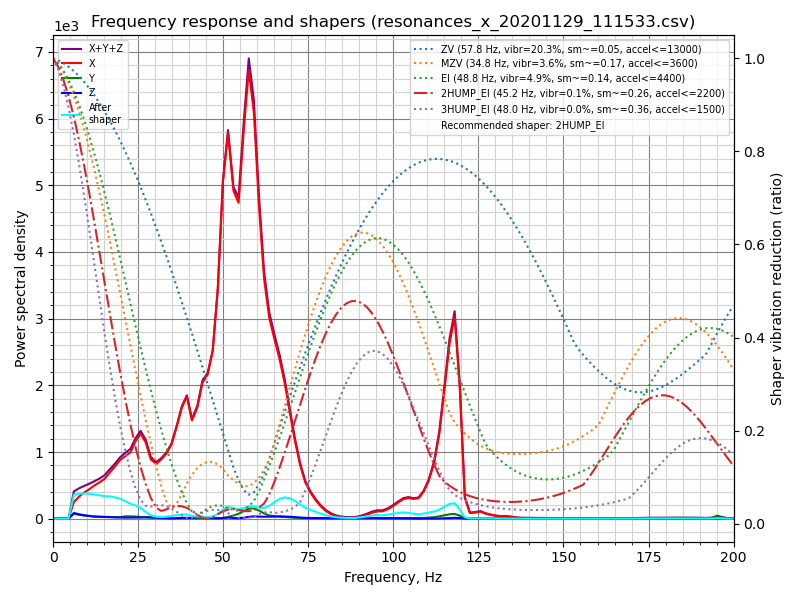``````Fitted shaper 'zv' frequency = 57.8 Hz (vibrations = 20.3%, smoothing ~= 0.053)
To avoid too much smoothing with 'zv', suggested max_accel <= 13000 mm/sec^2
Fitted shaper 'mzv' frequency = 34.8 Hz (vibrations = 3.6%, smoothing ~= 0.168)
To avoid too much smoothing with 'mzv', suggested max_accel <= 3600 mm/sec^2
Fitted shaper 'ei' frequency = 48.8 Hz (vibrations = 4.9%, smoothing ~= 0.135)
To avoid too much smoothing with 'ei', suggested max_accel <= 4400 mm/sec^2
Fitted shaper '2hump_ei' frequency = 45.2 Hz (vibrations = 0.1%, smoothing ~= 0.264)
To avoid too much smoothing with '2hump_ei', suggested max_accel <= 2200 mm/sec^2
Fitted shaper '3hump_ei' frequency = 48.0 Hz (vibrations = 0.0%, smoothing ~= 0.356)
To avoid too much smoothing with '3hump_ei', suggested max_accel <= 1500 mm/sec^2
Recommended shaper is 2hump_ei @ 45.2 Hz
``````

``````~/klipper/scripts/calibrate_shaper.py /tmp/resonances_x_*.csv -o /tmp/shaper_calibrate_x.png --max_smoothing=0.2
``````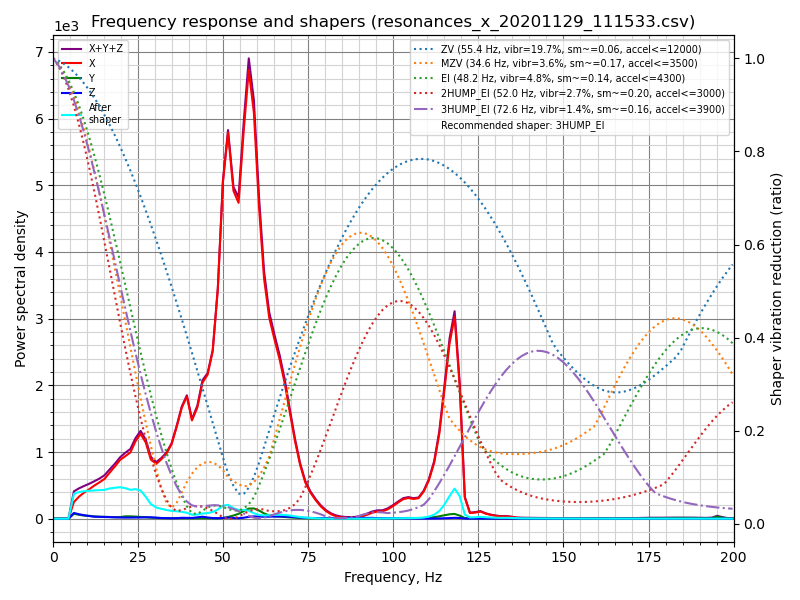``````Fitted shaper 'zv' frequency = 55.4 Hz (vibrations = 19.7%, smoothing ~= 0.057)
To avoid too much smoothing with 'zv', suggested max_accel <= 12000 mm/sec^2
Fitted shaper 'mzv' frequency = 34.6 Hz (vibrations = 3.6%, smoothing ~= 0.170)
To avoid too much smoothing with 'mzv', suggested max_accel <= 3500 mm/sec^2
Fitted shaper 'ei' frequency = 48.2 Hz (vibrations = 4.8%, smoothing ~= 0.139)
To avoid too much smoothing with 'ei', suggested max_accel <= 4300 mm/sec^2
Fitted shaper '2hump_ei' frequency = 52.0 Hz (vibrations = 2.7%, smoothing ~= 0.200)
To avoid too much smoothing with '2hump_ei', suggested max_accel <= 3000 mm/sec^2
Fitted shaper '3hump_ei' frequency = 72.6 Hz (vibrations = 1.4%, smoothing ~= 0.155)
To avoid too much smoothing with '3hump_ei', suggested max_accel <= 3900 mm/sec^2
Recommended shaper is 3hump_ei @ 72.6 Hz
``````

``````[resonance_tester]
accel_chip: ...
probe_points: ...
max_smoothing: 0.25  # an example
``````

### 測試自定義軸¶

`TEST_RESONANCES` 命令支持自定義軸。雖然這對於輸入整形器校準並不是很有用，但它可用於深入研究打印機共振並檢查皮帶張力等。

``````TEST_RESONANCES AXIS=1,1 OUTPUT=raw_data
TEST_RESONANCES AXIS=1,-1 OUTPUT=raw_data
``````

``````~/klipper/scripts/graph_accelerometer.py -c /tmp/raw_data_axis*.csv -o /tmp/resonances.png
``````

``````TEST_RESONANCES AXIS=0,1 OUTPUT=raw_data
TEST_RESONANCES AXIS=-0.866025404,-0.5 OUTPUT=raw_data
TEST_RESONANCES AXIS=0.866025404,-0.5 OUTPUT=raw_data
``````

``````~/klipper/scripts/graph_accelerometer.py -c /tmp/raw_data_axis*.csv -o /tmp/resonances.png
``````

## 輸入整形器自動校準¶

``````SHAPER_CALIBRATE
``````

``````Calculating the best input shaper parameters for y axis # 正在計算y軸的最佳輸入整形參數
Fitted shaper 'zv' frequency = 39.0 Hz (vibrations = 13.2%, smoothing ~= 0.105) # 擬合整形「zv」
To avoid too much smoothing with 'zv', suggested max_accel <= 5900 mm/sec^2 # 為避免使用「zv」方法產生過度平滑，建議最大加速度<=5900 mm/sec^2
Fitted shaper 'mzv' frequency = 36.8 Hz (vibrations = 1.7%, smoothing ~= 0.150) # 擬合整形「mzv」
To avoid too much smoothing with 'mzv', suggested max_accel <= 4000 mm/sec^2 # 為避免使用「mzv」方法產生過度平滑，建議最大加速度<=4000 mm/sec^2
Fitted shaper 'ei' frequency = 36.6 Hz (vibrations = 2.2%, smoothing ~= 0.240) # 擬合整形「ei」
To avoid too much smoothing with 'ei', suggested max_accel <= 2500 mm/sec^2 # 為避免使用「ei」方法產生過度平滑，建議最大加速度<=2500 mm/sec^2
Fitted shaper '2hump_ei' frequency = 48.0 Hz (vibrations = 0.0%, smoothing ~= 0.234) # 擬合整形「2hump_ei」
To avoid too much smoothing with '2hump_ei', suggested max_accel <= 2500 mm/sec^2 # 為避免使用「2hump_ei」方法產生過度平滑，建議最大加速度<=2500 mm/sec^2
Fitted shaper '3hump_ei' frequency = 59.0 Hz (vibrations = 0.0%, smoothing ~= 0.235) # 擬合整形「3hump_ei」
To avoid too much smoothing with '3hump_ei', suggested max_accel <= 2500 mm/sec^2 # 為避免使用「3hump_ei」方法產生過度平滑，建議最大加速度<=2500 mm/sec^2
Recommended shaper_type_y = mzv, shaper_freq_y = 36.8 Hz # 建議shaper_type_y = mzv, shaper_freq_y = 36.8 Hz
``````

``````SHAPER_CALIBRATE AXIS=Y
``````

### 輸入整形器重新校準¶

`SHAPER_CALIBRATE` 命令也可用於將來重新校準輸入整形器，尤其是在對打印機進行一些可能影響其運動學的更改時。可以使用“SHAPER_CALIBRATE”命令重新運行完整校準，或者通過提供“AXIS=”參數將自動校準限制在單個軸上，例如

``````SHAPER_CALIBRATE AXIS=X
``````

## 加速度計數據的離線處理¶

``````SET_INPUT_SHAPER SHAPER_FREQ_X=0 SHAPER_FREQ_Y=0
TEST_RESONANCES AXIS=X OUTPUT=raw_data
``````

• 繪製原始加速度計數據（使用 `-r` 參數），僅支持 1 個輸入;
• 繪製頻率響應（不需要額外的參數），如果指定了多個輸入，則計算平均頻率響應；
• 比較幾個輸入之間的頻率響應（使用“-c”參數）；您還可以通過“-a x”、“-a y”或“-a z”參數指定要考慮的加速度計軸（如果未指定，則使用所有軸的振動總和）；
• 繪製頻譜圖（使用 `-s` 參數），僅支持 1 個輸入；您還可以通過“-a x”、“-a y”或“-a z”參數指定要考慮的加速度計軸（如果未指定，則使用所有軸的振動總和）。

``````~/klipper/scripts/graph_accelerometer.py /tmp/raw_data_x_*.csv -o /tmp/resonances_x.png -c -a z
``````

shaper_calibrate.py 腳本接受 1 個或多個輸入，並且可以運行輸入整形器的自動調整，並建議適用於所有提供的輸入的最佳參數。它將建議的參數打印到控制台，如果提供了 `-o output.png` 參數，它還可以生成圖表，如果指定了 `-c output.csv` 參數，則可以生成 CSV 文件。

• 在第一次將加速度計連接到工具頭上，第二次將加速度計按順序連接到床身的情況下，在床吊具打印機上為單個軸運行兩次“TEST_RESONANCES AXIS=X OUTPUT=raw_data”（和“Y”軸）檢測軸交叉共振並嘗試使用輸入整形器消除它們。
• 在帶有玻璃床和磁性表面（更輕）的床吊具上運行兩次“TEST_RESONANCES AXIS=Y OUTPUT=raw_data”，以找到適用於任何打印表面配置的輸入整形器參數。
• 結合來自多個測試點的共振數據。
• 結合來自 2 軸的共振數據（例如，在床拋投打印機上配置 X 軸 input_shaper 從 X 軸和 Y 軸共振以消除的振動，以防噴嘴在 X 軸方向移動時“捕捉”打印）。